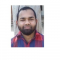## How to find the moving standard deviation in an R matrix?Published on 23-Nov-2021 06:52:55
To find the moving standard deviation in a matrix is done in the same way as in a data frame, we just need to use the matrix object name in place of data frame name. Hence, we can make use of rollapply function of zoo package for this purpose.For example, ... Read More

## How to round the summary output in R?Published on 23-Nov-2021 06:49:16
To round the output of summary function in R, we can use digits argument while applying the summary function.For example, if we have a data frame called df then to find the summary statistics with two digits in the output we can use the below given command −summary(df, digits=2)Example 1Following ... Read More

## How to find the row wise sum for n number of columns in R?Published on 23-Nov-2021 06:36:30
To find the row wise sum of n number of columns can be found by using the rowSums function along with subsetting of the columns with single square brackets.For example, if we have a data frame called df that contains five columns and we want to find the row sums ... Read More

## How to extract the maximum value from named vector in R?Published on 23-Nov-2021 06:31:08
To extract the maximum value from named vector in R, we can use which.max function.For example, if we have a vector called X which is a named vector then we can use the following command to find the maximum value in X.X[which.max(X)]Check out the below examples to understand how it ... Read More

## How to reduce the space between Y-axis value and ticks using ggplot2 in R?Published on 23-Nov-2021 06:21:07
To reduce the space between axis value and ticks using ggplot2, we can use theme function of ggplot2 package with margin set to 0.For example, if we have a data frame called df that contains two columns say x and y then the scatterplot between x and y with reduced ... Read More

## How to display fraction in base R plot?Published on 23-Nov-2021 06:15:23
To display fraction in base R plot, we can use frac function in text function.For example, if we create a plot of vectors called X and Y with display of over form fraction as X over Y at X=2 and Y= 2 then we can use the following code −text(2, ... Read More

## How to display fraction in a plot title using ggplot2 in R?Published on 23-Nov-2021 06:11:56
To display fraction in a plot title using ggplot2 in R, we can use frac function inside ggtitle function of ggplot2 package. Generally, fraction is displayed as X/Y but frac function helps to create the fraction in over form.Check out the below example to understand how over form of fraction ... Read More

## How to find the sequence of correlation between variables in an R data frame or matrix?Published on 23-Nov-2021 05:51:00
To find the sequence of correlation between variables in an R data frame or matrix, we can use correlate and stretch function from corrr package.For example, if we have a data frame called df then we can find the sequence of correlation between variables in df by using the below ... Read More

## How to create a matrix with vectors as elements in R?Published on 23-Nov-2021 05:45:59
To create a matrix with vectors as elements in R, we can create arrays because an array contains matrices with vectors as elements.Check out the below given examples of arrays and vectors extracted by the arrays to understand how matrices stored in an array represent vectors as elements.Example 1Following snippet ... Read More

## How to create a vertical line in a time series plot in base R?Published on 23-Nov-2021 05:42:07
To create a time series plot, we can use simply apply plot function on time series object and if we want to create a vertical line on that plot then abline function will be used with v argument.For example, if we have a time series object called T and we ... Read More